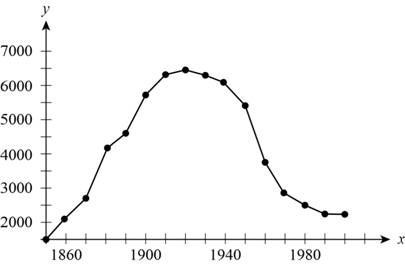# To estimate: The average rate of change in number of farm in between given interval, (i) 1860 and 1890 (ii) 1950 and 1970.### Precalculus: Mathematics for Calcu...

6th Edition
Stewart + 5 others
Publisher: Cengage Learning
ISBN: 9780840068071### Precalculus: Mathematics for Calcu...

6th Edition
Stewart + 5 others
Publisher: Cengage Learning
ISBN: 9780840068071

#### Solutions

Chapter 2.4, Problem 30E

(a)

To determine

## To estimate: The average rate of change in number of farm in between given interval,(i) 1860 and 1890(ii) 1950 and 1970.

Expert Solution

Rate of change in the number of farms between 1860 and 1890 is approximately 84Farms/year . and between 1950 and 1970 is 130Farms/year .

### Explanation of Solution

Given:

The given graph is,Figure (1)

Formula used:

Average rate of change of function y=f(x) between x=a and x=b .

Averagerateofchange=ChangeinyChangeinx=f(b)f(a)ba (1)

Calculation:

The graph indicates the number of farms in united states from 1850 to 2000.

(i) Rate of change in the number of farms between 1860 and 1890.

From the graph it can be noticed that the number of farms in united states in year 1860 is 2000 and in year 1890 is 4500

Substitute 2000 for f(a) , 4500 for f(b) , 1860 for a and 1890 for b in equation (1).

Averagerateofchange=4500200018901860=25003084Farms/year

The average rate of change of temperature of bowl of soup over the first 20 minutes is 4.05°F/min .

(ii) Rate of change in the number of farms between 1950 and 1970.

From the graph it can be noticed that the number of farms in united states in year 1950 is 5600 and in year 1970 is 3000

Substitute 5600 for f(a) , 3000 for f(b) , 1950 for a and 1970 for b in equation (1).

Averagerateofchange=3000560019701950=260020=130Farms/year

Therefore, rate of change in the number of farms between 1860 and 1890 is approximately 84Farms/year . and between 1950 and 1970 is 130Farms/year .

(b)

To determine

### To estimate: The decade in which the number of farm experience the greatest average rate of decline.

Expert Solution

The decade between 1950 to 1960 has the greatest average rate of decline.

### Explanation of Solution

Given:

The given graph is,Figure (1)

Calculation:

From the graph it can be easily determined that the decade between 1950 to 1960 has the greatest average rate of decline.

Calculate the average rate of decline in this interval to support the opinion.

It can be noticed that the number of farms in united states in year 1950 is 5600 and in year 1960 is 3700.

Substitute 5600 for f(a) , 3700 for f(b) , 1950 for a and 1960 for b in equation (1).

Averagerateofchange=3700560019601950=190010=190Farms/year

Hence, in the decade between 1950 to 1960, the greatest average rate of decline is observed.

### Have a homework question?

Subscribe to bartleby learn! Ask subject matter experts 30 homework questions each month. Plus, you’ll have access to millions of step-by-step textbook answers!